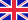•# Spectral Transform Methods

Autor: Davinia Chilton

A transform method developed by Fokas, is used for the study of two-point boundary value problems for linear evolution partial differential equations of arbitrary order, posed on a finite space domain. An appropriately defined x-differential operator, and... Viac o knihe

Na objednávku, dodanie 2-4 týždne

69.11 €

bežná cena: 81.30 €

## O knihe

A transform method developed by Fokas, is used for the study of two-point boundary value problems for linear evolution partial differential equations of arbitrary order, posed on a finite space domain. An appropriately defined x-differential operator, and suitable initial and boundary data are assumed. The solution representation is expressible as an integral in the complex plane. For problems of odd order such representations are new, while for even orders it is shown that they are equivalent to classical series representations. Spectral codes are developed for the numerical solution of a variety of illustrative examples, with many different types of boundary conditions. Finally, these codes are generalised and developed for linear third order problems for the solution of two-point boundary value problems for the nonlinear Korteweg-deVries equation.

• Vydavateľstvo: LAP Lambert Acad. Publ.
• Rok vydania: 2009
• Formát: Paperback
• Rozmer: 220 x 150 mm
• Jazyk: Anglický jazyk
• ISBN: 9783838320625

Generuje redakčný systém BUXUS CMS spoločnosti ui42.